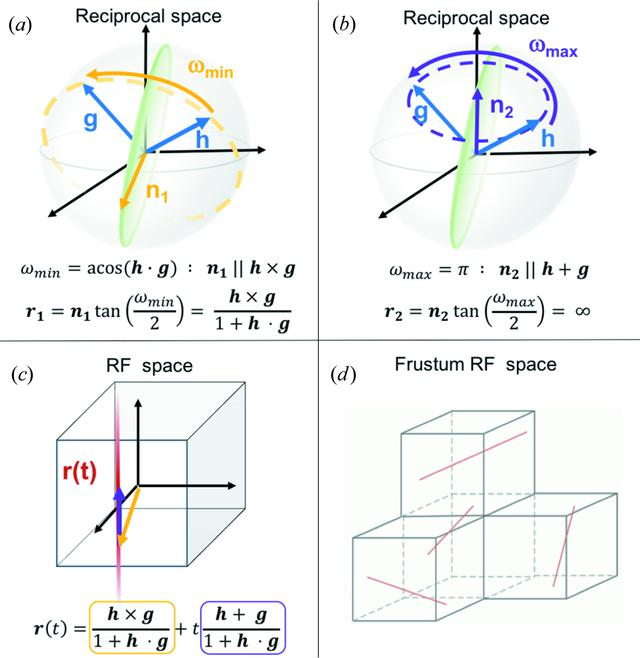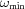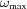disable zoom     view article Figure 1 (a), (b) Limiting cases of the rotation operations that bring a Bragg spot h onto an observed scattering vector g. The rotation axis requiring the minimum rotation,, is parallel to h × g, while that requiring the maximum rotation,, is parallel to h + g. All possible rotation axes must satisfy h · n = g · n and thus lie on the green circular plane that bisects the two vectors. (c) The full set of such rotations can be expressed as a linear combination of the two limiting cases, as given by the equation for r(t). From equation (1), these limiting cases map to vectors in RF space, and the expression for the geodesic, r(t), is an infinite straight line. (d) To avoid searching an infinite space, FELIX maps RF space into four frustums, shown as the four cubes in the image. All of the surfaces of the frustums are mathematically connected, so that a geodesic passing through one surface continues on in the neighboring frustum. The red lines shown in each frustum are then actually a single geodesic that is unbroken when the surface connectivity is applied.JOURNAL OFAPPLIEDCRYSTALLOGRAPHY
ISSN: 1600-5767
Volume 50| Part 4| August 2017| Pages 1075-1083
Follow J. Appl. Cryst.